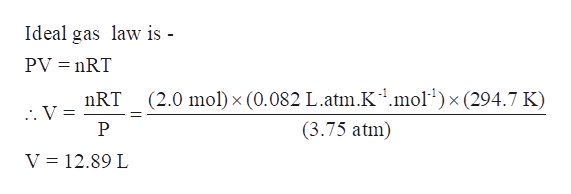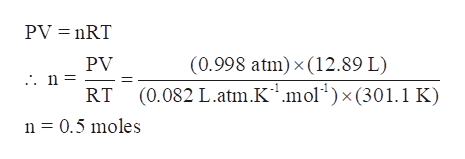# A glass container was initially charged with 2.00 moles of a gas sample at 3.75 atm and 21.7°C. Some of the gas was released as the temperature was increased to 28.1°C, so the final pressure in the container was reduced to 0.998 atm. Assume the volume stays the same. How many moles of the gas sample are present at the end?

Question
126 views

A glass container was initially charged with 2.00 moles of a gas sample at 3.75 atm and 21.7°C. Some of the gas was released as the temperature was increased to 28.1°C, so the final pressure in the container was reduced to 0.998 atm. Assume the volume stays the same. How many moles of the gas sample are present at the end?

check_circle

Step 1

A glass container was initially charged with 2.00 moles of a gas sample at 3.75 atm and 21.7°C. Some of the gas was released as the temperature was increased to 28.1°C, so the final pressure in the container was reduced to 0.998 atm. Assume the volume stays the same. The number moles of the gas sample are present at the end is to be determine.

Step 2

Calculate the volume occupied:

Assuming the gas to be ideal gas, volume occupied by the gas can be determined using the ideal gas law.

Given-

Moles of gas, n = 2.00 moles

Pressure, P = 3.75 atm

Temperature, T = 21.7°C = 21.7 + 273 = 294.7 K

Gas constant, R = 0.082 L.atm.K-1.mol-1help_outlineImage TranscriptioncloseIdeal gas law is - PV nRT (2.0 mol) x (0.082 L.atm.K1.mol)x (294.7 K) nRT . V = P (3.75 atm) V 12.89 L fullscreen
Step 3

Now, when some of the gas was released, its volume remains the same,

V = 12.89 L

T = 28.1 °C = 28.1 + 273 = 301.1 K

P = 0.998 atm

Again ideal gas law can be used to determine the moles of gas left after releasing gas.

...help_outlineImage TranscriptionclosePV nRT PV (0.998 atm) x (12.89 L) (0.082 L.atm.K1 mol RT x 301.1 K) n 0.5 moles fullscreen

### Want to see the full answer?

See Solution

#### Want to see this answer and more?

Solutions are written by subject experts who are available 24/7. Questions are typically answered within 1 hour.*

See Solution
*Response times may vary by subject and question.
Tagged in

### Gas laws